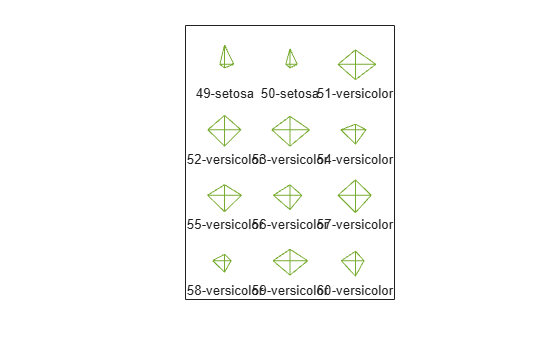Main Content

# glyphplot

## Syntax

```glyphplot(X) glyphplot(X,'glyph','face') glyphplot(X,'glyph','face','features',f) glyphplot(X,...,'grid',[rows,cols]) glyphplot(X,...,'grid',[rows,cols],'page',p) glyphplot(X,...,'centers',C) glyphplot(X,...,'centers',C,'radius',r) glyphplot(X,...,'obslabels',labels) glyphplot(X,...,'standardize',method) glyphplot(X,...,prop1,val1,...) h = glyphplot(X,...) ```

## Description

`glyphplot(X)` creates a star plot from the multivariate data in the n-by-p matrix `X`. Rows of `X` correspond to observations, columns to variables. A star plot represents each observation as a “star” whose ith spoke is proportional in length to the ith coordinate of that observation. `glyphplot` standardizes `X` by shifting and scaling each column separately onto the interval `[0,1]` before making the plot, and centers the glyphs on a rectangular grid that is as close to square as possible. `glyphplot` treats `NaN`s in `X` as missing values, and does not plot the corresponding rows of `X`. `glyphplot(X,'glyph','star')` is a synonym for `glyphplot(X)`.

`glyphplot(X,'glyph','face')` creates a face plot from `X`. A face plot represents each observation as a “face,” whose ith facial feature is drawn with a characteristic proportional to the ith coordinate of that observation. The features are described in Face Features.

`glyphplot(X,'glyph','face','features',f)` creates a face plot where the ith element of the index vector `f` defines which facial feature will represent the ith column of `X`. `f` must contain integers from `0` to `17`, where `0` indicate that the corresponding column of `X` should not be plotted. See Face Features for more information.

`glyphplot(X,...,'grid',[rows,cols])` organizes the glyphs into a `rows`-by-`cols` grid.

`glyphplot(X,...,'grid',[rows,cols],'page',p)` organizes the glyph into one or more pages of a `rows`-by-`cols` grid, and displays the page `p`. If `p` is a vector, `glyphplot` displays multiple pages in succession. If `p` is `'all'`, `glyphplot` displays all pages. If `p` is `'scroll'`, `glyphplot` displays a single plot with a scrollbar.

`glyphplot(X,...,'centers',C)` creates a plot with each glyph centered at the locations in the n-by-2 matrix `C`.

`glyphplot(X,...,'centers',C,'radius',r)` creates a plot with glyphs positioned using `C`, and scale the glyphs so the largest has radius `r`.

`glyphplot(X,...,'obslabels',labels)` labels each glyph with the text in `labels`. By default, the glyphs are labelled `1:N`. Use `''` for blank labels.

`glyphplot(X,...,'standardize',method)` standardizes `X` before making the plot. Choices for `method` are

• `'column'` — Maps each column of `X` separately onto the interval [0,1]. This is the default.

• `'matrix'` — Maps the entire matrix `X` onto the interval `[0,1]`.

• `'PCA'` — Transforms `X` to its principal component scores, in order of decreasing eigenvalue, and maps each one onto the interval `[0,1]`.

• `'off'` — No standardization. Negative values in `X` may make a star plot uninterpretable.

`glyphplot(X,...,prop1,val1,...)` sets properties to the specified property values for all line graphics objects created by `glyphplot`.

`h = glyphplot(X,...)` returns a matrix of handles to the graphics objects created by `glyphplot`. For a star plot, `h(:,1)` and `h(:,2)` contain handles to the line objects for each star's perimeter and spokes, respectively. For a face plot, `h(:,1)` and `h(:,2)` contain object handles to the lines making up each face and to the pupils, respectively. `h(:,3)` contains handles to the text objects for the labels, if present.

### Face Features

The following table describes the correspondence between the columns of the vector `f`, the value of the `'Features'` input parameter, and the facial features of the glyph plot. If `X` has fewer than 17 columns, unused features are displayed at their default value.

ColumnFacial Feature

1

Size of face

2

Forehead/jaw relative arc length

3

Shape of forehead

4

Shape of jaw

5

Width between eyes

6

Vertical position of eyes

7

Height of eyes

8

Width of eyes (this also affects eyebrow width)

9

Angle of eyes (this also affects eyebrow angle)

10

Vertical position of eyebrows

11

Width of eyebrows (relative to eyes)

12

Angle of eyebrows (relative to eyes)

13

Direction of pupils

14

Length of nose

15

Vertical position of mouth

16

Shape of mouth

17

Mouth arc length

## Examples

collapse all

Load the sample data.

```load carsmall X = [Acceleration Displacement Horsepower MPG Weight];```

Create a star plot of the data in `X` . Standardize the data before plotting.

```glyphplot(X,'standardize','column','obslabels',Model,'grid',[2 2],... 'page','scroll');```Create a face plot of the data in `X` .

`glyphplot(X,'glyph','face','obslabels',Model,'grid',[2 3],'page',9);`## See Also

Introduced before R2006a

Download ebook